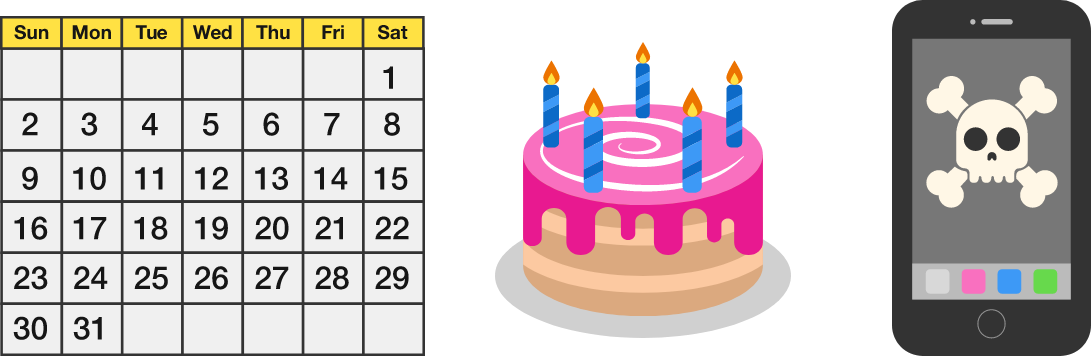### Number Theory

What is the last digit of the result of the multiplication below? $123 \times 321$

Hint: There is no need to completely multiply these numbers.

# Last Digits

All of the problems in this quiz can be solved without completing full calculations if you focus in on just the part that matters. This kind of shortcut-seeking is a major skill you'll practice and explore as you learn number theory.

Some of the number theory tricks shortcuts in this course are purely theoretical, and others can be applied to doing anything from rapidly computing what day of the week your $100^\text{th}$ birthday will fall on to hacking smartphone software!# Last Digits

What is the last digit of $1 \times 2 \times 3 \times 4 \times 5 \times 6 \times 7 \times 8 \times 9\, ?$

# Last Digits

What is the last digit of $1 \times 3 \times 5 \times 7 \times 9 \times 11 \times 13 \, ?$

# Last Digits

What is the last digit of $2^{13} ?$

Hint: \begin{aligned} &2^1 = &\color{#D61F06}2\\ &2^2 = &\color{#D61F06}4\\ &2^3 = &\color{#D61F06}8\\ &2^4 = &1\color{#D61F06}6\\ &2^5 = &3\color{#D61F06}2\\ &2^6 = &6\color{#D61F06}4\\ &2^7 = &12\color{#D61F06}8\\ &2^8 = &25\color{#D61F06}6 \end{aligned}

# Last Digits

What is the last digit of $6^{789} ?$

Hint:

• $6 \times 6 = 3\color{#D61F06}6$
• $36 \times 6 = 21\color{#D61F06}6$

×